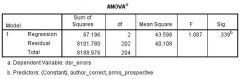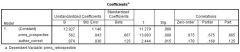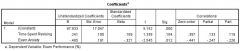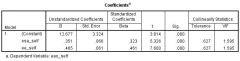• Shuffle
Toggle On
Toggle Off
• Alphabetize
Toggle On
Toggle Off
• Front First
Toggle On
Toggle Off
• Both Sides
Toggle On
Toggle Off
Toggle On
Toggle Off
Front

### How to study your flashcards.

Right/Left arrow keys: Navigate between flashcards.right arrow keyleft arrow key

Up/Down arrow keys: Flip the card between the front and back.down keyup key

H key: Show hint (3rd side).h key

A key: Read text to speech.a keyPlay buttonPlay buttonProgress

1/63

Click to flip

### 63 Cards in this Set

• Front
• Back
 A one _____ unit increase in X is associated with a ‘standardized beta weight’ standard deviation % increase in Y standard deviation A researcher obtains a standardized beta weight of .50. The dependent variable has a standard deviation of 20.0. How much of an increase in the dependent variable would be expected based on a one standard deviation unit increase in the independent variable? 10 At the very least, a multiple regression has ___ independent variables. two In multiple regression, a dependent variable should be measured on _____ scale. interval/ratio If the dependent variable in a multiple regression is measured on an ordinal scale, it should have at least ______ levels. five In regression, independent variables such as gender are known to be ____ coded. dummy In regression, when an independent variable is measured on a nominal scale with three or more levels, one must use ____ coding. effect With respect to the regression equation, the only real difference between bivariate regression and multiple regression is that the equation includes more ____. beta weights. In multiple regression, a beta weight represents the independent variable’s _____ to the regression equation. unique contribution Beta weights in multiple regression are ___ correlations. not Pearson For the purposes of interpretation and comparison, it is typically more useful to consult the ____ beta values. standardized Standardized beta weights usually take on values between _____ -.99 to .99. In multiple regression, R represents the correlation between the values ____ by the model and the ____ values associated with the dependent variable. predicted; observed Adjusted R2 is correlated positively with ____ and negatively with _____. sample size; number of predictors (2 marks) In forced entry multiple regression, ___ of the independent variables are entered into the model. all In hierarchical multiple regression, the _____ decides the order in which independent variables are entered into the model. experimenter In _____, independent variables are included in the model based on a statistical algorithm. stepwise multiple regression Prior to conducting a multiple regression analysis, it is always good practice to examine the _____ between the variables. Pearson correlations In order to conduct a multiple regression analysis in SPSS within the menu options, one must use the _____ utility. Regression -> LinearBased on the SPSS output below, is the Model R value statistically significant? No, p = .339Based on the SPSS output below, which of the two variables is contributing more to the equation? prmq_prospective b1= .241; So, as time spent revising increases by ____, exam performance increases by .241. one unit b2= -.485; So, as exam anxiety increases by one unit, exam performance _____ by -.485. decreases b1= .169; So, as time spent revising increases by one standard deviation, exam performance increases by ____ of a standard deviation. 16.9% ______ can be estimated by calculating the difference between the predicted dependent variable scores and the observed dependent variable scores. Residuals Beta weights cannot be squared for the purposes of speaking of percentage of variance account for; however, the corresponding ______ can be squared for such a purpose. semi-partial correlations A semi-partial correlation represents the _____ between an independent variable and dependent variable, controlling for the effects of _____ on the independent variable. unique association; another variableIn the below SPSS output, what is the semi-partial correlation between Exam Anxiety and Exam Performance? -.226In the SPSS output below, what is the p value associated with the Time Spent Revising semi-partial correlation? .184 There are two categories of evaluating the quality of a model that has been estimated via multiple regression: ____ and ____. accuracy; generalizability (2 marks) There are two ways to evaluate the accuracy of a multiple regression model: ____ and ____. residual outliers; influential cases (2 marks) In the context of regression, an outlier is an observation that is especially poorly _____ by the model, in comparison to the other observations in the sample of data. predicted To some degree, the evaluation of residual outliers can be done qualitatively through a ____. scatter plot. Regression outliers can be evaluated statistically through the use of … standardized residuals. In a perfectly normal distribution of residuals, 99% of standardized residuals should lie between … ± 2.5 Any case for which the absolute value of the standardized residual is ____ or more, is likely to be an outlier. 3.0 If the distribution of regression residuals is perfectly normal, and there are actually no outlying standardized residuals, one would still expect ____ standardized residuals to be associated with a value of |3.0| or greater in a sample of 1000 observations. 2.7 In SPSS, the process of evaluating whether there are any regression residuals involves first ____ the residuals and then plotting them in a … saving; histogram Influential cases have a big impact on the value of… multiple R In this unit, influential cases were evaluated based on ____ values. Cook's Distance According to Weisberg (1982), Cook’s Distance values greater than ____ should be considered influential cases. one In SPSS, in order to obtain the Cook’s Distance values, you need to use the ____ utility in regression. Save... When Cook’s Distance values are produced in SPSS, they are labelled ____ in SPSS data file. COO_1 When we run regression, we hope to be able to _____ the sample model to the entire population. generalize The independence assumption in statistics implies that all outcome values were derived from ____. different people. The more advanced assumptions associated with multiple regression are: no multicollinearity; homoscedasticity; independent errors; normally distributed errors (4 marks) _____ is observed when two predictor variables are highly inter-correlated (e.g., .95). Collinearity Collinearity can be assessed by examining the Pearson correlations between the _____ variables. independent ______ is observed when a predictor variable shares a substantial amount of variance with a series of other independent variables. Multicollinearity Multicollinearity can be assessed by examining two diagnostics known as _____ and the ____. Tolerance; Variance Inflation Factor Tolerance is equal to_____, where the independent variable is regressed onto the other independent variables. 1 – R2 _____ represents the percentage of variance in the independent variable that is unique, i.e., that is not shared or accounted for by the other independent variables. Tolerance Tolerance values are calculated for each _____ included in the analysis. independent variable The Variance Inflation Factor is directly correlated with Tolerance by a function of … 1 / Tolerance The Variance Inflation Factor tells us the degree to which the _____ associated with the beta weights are ____ than they would otherwise be in the case where there was the total absence of multicollinearity. standard errors; larger (2 marks) Greater tolerance levels indicate ____ levels of multicollinearity. lesser ______ VIF levels indicate lesser levels of multicollinearity. Lower Tolerance levels as low as _____ should be considered acceptable. .10 VIF levels no larger than _____ should be considered acceptable. 10 In SPSS, in order to get the Tolerance and VIF estimates, one must press the _____ button and select the _____ option. Statistics…; Collinearity diagnostics (2 marks)According to the SPSS output below, which values indicate to someone whether the independent variables may share an excess amount of variance with each other? .627; 1.595 (1 mark) High levels of multicollinearity suggest that one or more of the independent variables is/are … redundant. In SPSS, in addition to looking at the histogram of standardized residuals, regression outliers can be examined via the … Normal Probability Plot.